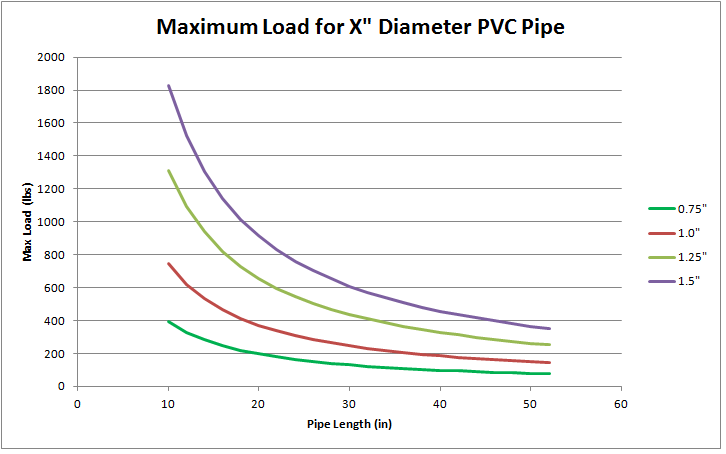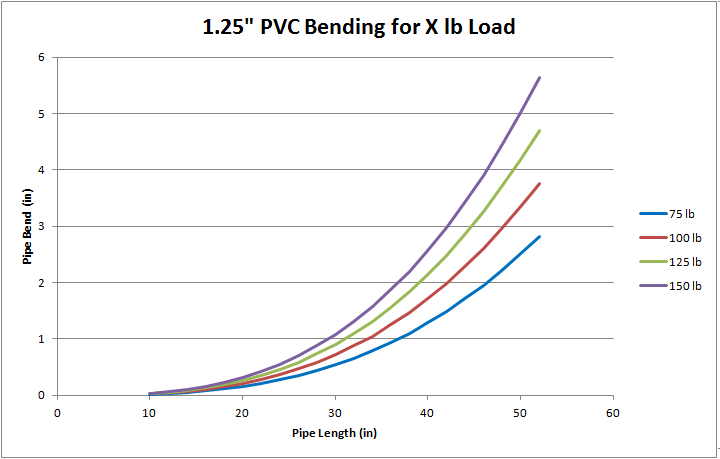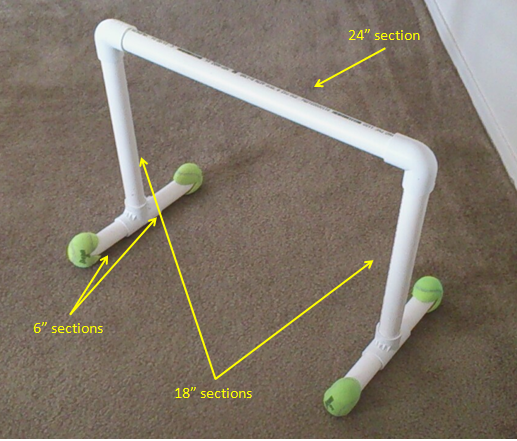# DIY Parallette Bars Using PVC: An Engineering Approach

Parallette bars are a cheap and easy way to upgrade a home workout routine. There are a number of great bodyweight exercises you can do with them, and they are light and portable, so you can take them anywhere you want.

I first discovered parallette bars when browsing through the Buff Dudes Youtube channel, where they provide a handy how-to video that I embedded for your convenience:

The project looked pretty simple, so I decided I would make some myself. Upon going to the hardware store, however, I found that the steel pipe they referenced in the video was a tad pricey and unfortunately the store didn't have all the quantities needed. Defeated, I went back home to sulk at my failed attempt at an easy DIY project.

I started thinking, though, what if I built the bars with less expensive PVC pipe? PVC has a lot of good qualities: it is light, cheap, and can be pieced together easily and quickly. It is also pretty flexible, so it can bend quite a bit before breaking. Of course, this led to the obvious conundrum, can it support my weight safely? The 3/4" steel used for the Buff Dudes project certainly looked sturdy, so that would definitely be the best bet, but I wondered...with a bit of know-how, could I construct some PVC parallettes that would still be sturdy and safe, but also cheap?

Time to Crunch Some Numbers with PVC

I had to get rid of some of the cobwebs in my brain and remember some of the things from the Statics course I took in college, but after some good old fashioned internet searches I was able to find the equations I needed. The problem is basically that of a straight beam supported on two sides, with a force placed in the middle. That force represents your hand holding the bar. Basically, each bar has to support half your body weight (since there are two), but it is of course better to design them so that they support much more than that (for safety).The beam of length L represents the PVC pipe, and F is the force pushing down on the pipe where your hands would be holding it.

Warning: math content follows. If you aren't too savvy in that area, you can scroll down to the graphs for the answers. If you are savvy in that area, let me know if I made a mistake.

So, basic statics problem: the sum of the forces and moments must add up to zero.

ΣF = A + B - F = 0     (1)

ΣM = B*L - F*L/2 = 0     (2)

Solving for B gives B = F/2, and since A + B = F, A must equal F/2 as well.

So we can see that each vertical PVC pipe must support half the applied force, makes sense. The maximum moment is equal to F*L/4, since there are two supports and each contributes evenly, it halves the moment F*L/2, giving F*L/4.

Now to plug this result into the equation for allowable normal stress:

σallow = ( Mmax * y ) / I     (3)

Where σallow is the maximum normal stress (in units of pressure), Mmax is the maximum moment in the normal direction (i.e. perpendicular to the beam, in units of length*force), y is the height of the beam (or radius of the PVC pipe in our case), and I is the moment of inertia (in units of length^4).

I found some stat sheets for PVC that gave the maximum allowable stress to be equal to around 14,000 PSI. We already found the maximum moment to be F*L/4, and the y component is just the outer radius of the PVC pipe. The only factor left is the moment of inertia, which, for a hollow cylinder, is:

Icylinder = (π/4)*(ro­ - ri4)     (4)

Where ro is the outer radius of the pipe and ri is the inner radius of the pipe. So, to find the maximum force, we simply plug in F*L/4 for Mmax and move some things around in (3) to get:

Fmax = 4*σallow * I / (L * ro)     (5)

Now we have a design equation with two degrees of freedom! We can vary either the length of the pipe or the diameter and see what the maximum force is!

It is also perhaps more useful to know the bending of the pipe as well as its breaking point. PVC can bend quite a bit before it breaks, and if you are holding on to something that is bending and wobbling around, that's not exactly good either.

To calculate the deflection, I used the formula:

y = F*L3 / ( 48*E*I)

Where y is the deflection, and E is the modulus of elasticity, which is 40,000 psi for PVC.

Enough Math, Here Are the Graphs

First, here is a graph showing the maximum load of PVC for varying lengths and diameters:Based on the above graph, I decided that a 1.25" diameter PVC would be more than acceptable for me, and that a 24 in length (the one specified by the Buff Dudes) would be fine. Remember that each bar is, for most exercises,holding just half your weight.

I then took a look at the bending distance vs. length for the 1.25" diameter PVC to see if the 24 inch length would indeed be alright:At about the 24 inch mark in the above graph, loads up to 150 lbs (so, up to a 300 lb person) are supported pretty well, with about 0.5" of bending. Since I only weight about 150 lbs (meaning each bar would have to support 75 lbs), I thought the 1.25" diameter bar would be quite sufficient. I did, however, want to incorporate a fair amount of margin in the design, to compensate for the pipes becoming more flexible with age, as well as if anyone heavier than me wanted to use them.

Test Results

For fabrication, I followed the length guidelines set by the Buff Dudes for their bars, I will repeat them here:

Per Bar (so double it if you want two):

1 x 24" pipe
2 x 18" pipe
4 x 6" pipe
2 x elbow connectors
2 x tee connectors

I went to the local hardware store and picked up all the PVC needed (it comes out to 14' of pipe, and they are sold in 10' sections, so to make things easier I bought two 2' sections and one 10' section). Everything cost around \$20 total, which isn't too bad if you ask me.

Then, you just piece it all together:As you can see, I added tennis balls to the ends of the supports for added stability. Since I am an avid tennis player, I had a bunch of old balls around that I used for this, so it didn't add to the cost at all.

So how did the bars fare?

Well, the first noticeable thing is that the bottom supports tend to rotate with use, so that's a bit annoying. They can also wobble if you rock back and forth on the bars at all, so use caution. Always make sure that your weight is being loaded straight down on the bars, without any forces outward or inward. I think it kind of adds to the challenge, but it is something that makes the PVC inferior to steel pipe.

A way to improve these would be to add two more 24" sections to each bar at the base, so that the base would be better supported and not rotate. This would of course add some extra cost, but the safety benefit would be worth it.

EDIT: After a few months of use I did take a tumble at one point when the base sections torqued to the side just enough to topple. For that reason, I would STRONGLY recommend adding two 24" sections on each side for a solid base.

As far as bending goes, I don't notice any bend in the pipes when I use them, so I'd say the calculations were accurate, if not a bit too conservative.

Conclusion

If you want some low cost, light, strong, and easily assembled/disassembled parallette bars, then I assert that PVC is an acceptable material. Use caution when exercising with them, and make sure that the pipes are secure and snug in the connectors when assembling them. Also, if you don't want to disassemble the bars for transport, I recommend using a PVC cement at the joints for added safety.

If you want some ideas for exercises to do with them, here is a video from the Buff Dudes:

References

http://www.engineeringtoolbox.com/beam-stress-deflection-d_1312.html

http://www.engineersedge.com/beam_bending/beam_bending2.htm

http://www.professionalplastics.com/professionalplastics/PVCPipeSpecifications.pdf

http://emweb.unl.edu/Mechanics-Pages/Tan/Tan.htm